When I first examined the movies I just played them like a video. But I soon found there was much more to help me. There was text to look at. But most usefully I was able to step through a bit at a time and see what was happening on the graph.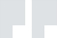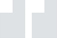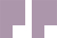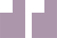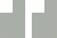The LabRat is daft but it can help.
When I was examining the movies I thought that speed had something to do with it. But that wasn't the whole story.
Crashing cars is so violent!
Size has a lot to do with this.
Because I'm mathematical I found the graphs in playback were most useful.
The bigger it is the harder it is to move.The way to approach this experiment is very systematically. First of all choose one object. Then alter either the force or the mass but keep the other one the same. Work through a number of combinations and see what happens to the velocity. The final answer is not the velocity itself but it is related to the velocity and how it changes.
I'd been getting confused by the effects of friction and air resistance. Once I turned them off I began to get some idea about what was happening. Then I could turn friction on again.Without friction the results with the different shapes were the same but with friction turned on they were very different.Ah! Force, mass and movement - they're all connected.I had to be a good scientist to make sense of this. It does not make sense if you just do one experiment or a few random experiments. You've got to work logically through a number of combinations. Then it's obvious!"I'm not mathematical, but there aren't that many combinations to try in this equation. When I sat down for a bit I was able to find an equation that related force and mass - but the third factor in the equation took a bit of working out because it was not simply velocity.The experiment with the crashing cars does illustrate the same rule - the same "Law" - but the opposite way round. There is still the same relation between force, mass and velocity - but this time the object is slowing down rather than speeding up.Second Law
Clues